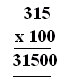Name: ___________________Date:___________________

 Email us to get an instant 20% discount on highly effective K-12 Math & English kwizNET Programs!

### Grade 3 - Mathematics9.10 Multiplication Review TestName: ___________________Date:___________________

### Grade 3 - Mathematics9.10 Multiplication Review Test

 Q 1: 1453 x 6Answer: Q 2: 13 x 4 = Answer: Q 3: In the multiplication problem shown what are the fators?315 and 100100 and 31500315 and 3150031500 Q 4: 123 x 2 = Answer: Q 5: In the multiplication problem shown what is the product?15636120 Q 6: 342 x 4Answer: Q 7: 375 x 3Answer: Q 8: 2309 x 6Answer: Question 9: This question is available to subscribers only! Question 10: This question is available to subscribers only!